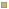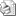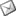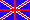HomeMathematicsWhich kind of mathematics for Quantum Mechanics? A survey and a program of research.
Date Display
Saturday, 23 September 2023
Last News
Correlated Article
History papers
Last twenty
 Which kind of mathematics for Quantum Mechanics? A survey and a program of research.## Which kind of mathematics for Quantum Mechanics?

### A survey and a program of research.##### Antonino DragoDept. of Physical Sciences, Univ. "Federico II", NaplesIn previous papers I reviewed past contributions untill to 1970 to the debate about the relationship of theoretical physics (=TP) with classical mathematics (=CM) which notoriously includes actual infinity (e.g. Zermelo's axiom).

Abstract

In previous papers I reviewed past contributions untill to 1970 to the debate about the relationship of theoretical physics (=TP) with classical mathematics (=CM) which notoriously includes actual infinity (e.g. Zermelo's axiom).
In this communication I will consider the contributions occurred after 1970 for suggesting a new kinds of mathematics more suitable for TP. Several strategies have been followed: 1) to deny a real role to mathematics in TP; 2) to enquire about a suitable formalisation of quantum logic for suggesting new mathematical tools ; 3) to suggest non-standard-analysis ; 4) to interpret the whole TP by means of set theory of Suppes' axiomatics; 5) to develop chaos theory; 6) to suggest Weyl's elementary mathematics (=WEM); 7) to inquire in CM by means of reverse mathematics which mathematical axioms PT needs; 8) to suggest constructive mathematics (=CoM); 9) to bound mathematics to discrete fields; 10) to simulate experimental physics and TP by means of computable objects, e.g. cellular automata; 11) to bound TP by means of some restriction of physical nature like finitism, or operativism, or essential inaccuracy. Then, I will consider the well-qualified mathematical strategies only, i.e. the no.s 3, 6, 7, 8 and 9. Furtherly, I will chose strategies no.s 6, 7 and 8 in order to consider the lesser powerful kind of mathematics which covers PT's needs.

CoM was by some authors - myself included - applied CoM to classical physics. All they evodentiated undecidable results; in particular Pour-El and Richards proved that some singular solutions of wave equations have not counterparts in CoM. I interpreted such undecidabilities as pertaining not to the mathematics of the physical theory at issue, but to which particular formulation of the theory is investigated. Hence, a successful introduction of CoM in a physical theory amounts to solve the problem of discovering among the past formulations of it a constructive one, or at most to invent a new formulation according to CoM. For ex., I proved that whereas Newton's formulation of mechanics includes undecidable principles, L. Carnot' formulation (1783) is free of undecidabilities. In thermodynamics, the old, "phenomenological" formulation is constructive, while Carathéodory's one does not.

However, is commonly held that modern TP - in particular quantum mechanics (=QM) -, represents an incomparable level of sophistication in the foundations of TP; hence, the problem is referred by most people to this theory. In the last years, an inconclusive debate took place among Bridges, Hellmann and da Costa. Since, in my opinion, the undecidable problems pertain to the particular formulation of QM one analyses, in order to obtain a positive result one has to search among the past formulations one of them which is in agreement with CoM. A modern version of Heisenberg's formulation was discovered, yet under the hypothesis that continuous spectra are constructively representable. Actually, constructive algebra is not well defined at present time.

One more suggestion is to investigate a QM formulation based upon symmetry. Actually in 1928 Weyl first introduced group theory in QM in a mathematical framework which may be recognised as WEM. It is a relevant fact that reverse mathematics characterises WEM at the lower levels of his hiearchy of kinds of ever powerful mathematics. Nevertheless in 1988 Feferman guessed that WEM is able to cover all mathematical need of TP. I instead proved that WEM covers a part only of TP. I discovered that WEM fits Cavalieri's and Torricelli's mathematics of indivisibles so that they have to be credited as the first inventors of calculus, yet in WEM version. Moreover, WEM supported the first statement of inertia principle in Cavalieri (1632 and then Torricelli in 1644, the same year of Descartes' statement). That proves that since their beginnings the whole mathematics and the whole TP severed in several foundations.
Under the light of reverse mathematics, an analysis follows on Weyl's book founding new mathematical foundations of QM in order to decide which Weyl's results pertain to respectively CoM, WEM, rigorous and non-standard analysis

Info

Drago A.: Which kind of mathematics for quantum mechanics? A survey and a program of research, In proceedings Conference SILFS, Urbino, 1999, in press